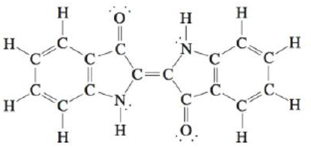# Indigo is the dye used in coloring blue jeans. The term navy blue is derived from the use of indigo to dye British naval uniforms in the eighteenth century. The structure of the indigo molecule is a. How many σ bonds and π bonds exist in the molecule? b. What hybrid orbitals are used by the carbon atoms in the indigo molecule?### Chemistry: An Atoms First Approach

2nd Edition
Steven S. Zumdahl + 1 other
Publisher: Cengage Learning
ISBN: 9781305079243

#### Solutions

Chapter
Section### Chemistry: An Atoms First Approach

2nd Edition
Steven S. Zumdahl + 1 other
Publisher: Cengage Learning
ISBN: 9781305079243
Chapter 4, Problem 53E
Textbook Problem
40 views

## Indigo is the dye used in coloring blue jeans. The term navy blue is derived from the use of indigo to dye British naval uniforms in the eighteenth century. The structure of the indigo molecule isa. How many σ bonds and π bonds exist in the molecule?b. What hybrid orbitals are used by the carbon atoms in the indigo molecule?

(a)

Interpretation Introduction

Interpretation: The structure of an indigo molecule has been given. Based on the structure, the stated questions have to be answered.

Concept introduction: The hybridization of an atom can be obtained by finding its steric number. The sum of the numbers of atoms bonded to the required atom and the number of lone pairs the atom has is known as the steric number.

If the steric number is 4 , the atom is sp3 hybridized.

If the steric number is 3 , the atom is sp2 hybridized.

If the steric number is 2 , the atom is sp hybridized.

Sigma bonds are the single bonds present between atoms in a compound. The second bonding that occurs between the atoms is known as the pi bonding.

To determine: The number of σ bonds and the number of π bonds present in the given structure.

### Explanation of Solution

The single bonds present in the molecular structure are known as σ bonds, whereas the second bond that occurs between atoms is referred to as a π bond.

A double bond is made up of one σ bond and one π bond.

The given structure of the compound is,

(b)

Interpretation Introduction

Interpretation: The structure of an indigo molecule has been given. Based on the structure, the stated questions have to be answered.

Concept introduction: The hybridization of an atom can be obtained by finding its steric number. The sum of the numbers of atoms bonded to the required atom and the number of lone pairs the atom has is known as the steric number.

If the steric number is 4 , the atom is sp3 hybridized.

If the steric number is 3 , the atom is sp2 hybridized.

If the steric number is 2 , the atom is sp hybridized.

Sigma bonds are the single bonds present between atoms in a compound. The second bonding that occurs between the atoms is known as the pi bonding.

To determine: The hybridization of the carbon atom in the given molecule.

### Still sussing out bartleby?

Check out a sample textbook solution.

See a sample solution

#### The Solution to Your Study Problems

Bartleby provides explanations to thousands of textbook problems written by our experts, many with advanced degrees!

Get Started

Find more solutions based on key concepts
Which of the following is fare) rich source(s) of vitamin E7 raw vegetable oil colorful foods, such as carrots ...

Nutrition: Concepts and Controversies - Standalone book (MindTap Course List)

22-12 Why does glycine have no D or L form?

Introduction to General, Organic and Biochemistry

Velocity is a(n) ___ quantity. (2.2)

An Introduction to Physical Science

What is the maximum number of bonds that a carbon atom can form?

Biology: The Dynamic Science (MindTap Course List)

What happens to the amplitude of the induced emf when the rate of rotation of a generator coil is doubled? (a) ...

Physics for Scientists and Engineers, Technology Update (No access codes included)

Write the molecular formula of butane. What is the name of C10H22.

Introductory Chemistry: An Active Learning Approach